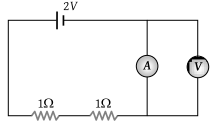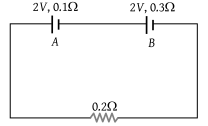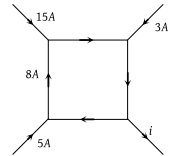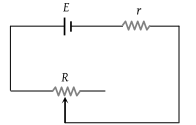In the circuit shown, A and V are ideal ammeter and voltmeter respectively. Reading of the voltmeter will be(1) 2 V

(2) 1 V

(3) 0.5 V

(4) Zero

Concept Questions :-

Kirchoff's voltage law
High Yielding Test Series + Question Bank - NEET 2020

Difficulty Level:

The terminal potential difference of a cell when short-circuited is (E = E.M.F. of the cell)

(1) E

(2) E/2

(3) Zero

(4) E/3

Concept Questions :-

Emf and terminal voltage
High Yielding Test Series + Question Bank - NEET 2020

Difficulty Level:

The potential difference in open circuit for a cell is 2.2 volts. When a 4-ohm resistor is connected between its two electrodes the potential difference becomes 2 volts. The internal resistance of the cell will be :

(1) 1 ohm

(2) 0.2 ohm

(3) 2.5 ohm

(4) 0.4 ohm

Concept Questions :-

Emf and terminal voltage
High Yielding Test Series + Question Bank - NEET 2020

Difficulty Level:

A cell whose e.m.f. is 2 V and internal resistance is 0.1 Ω, is connected with a resistance of 3.9 Ω. The voltage across the cell terminal will be :

(1) 0.50 V

(2) 1.90 V

(3) 1.95 V

(4) 2.00 V

Concept Questions :-

Emf and terminal voltage
High Yielding Test Series + Question Bank - NEET 2020

Difficulty Level:

n identical cells each of e.m.f. E and internal resistance r are connected in series. An external resistance R is connected in series to this combination. The current through R is

(1) $\frac{nE}{R+nr}$

(2) $\frac{nE}{nR+r}$

(3) $\frac{E}{R+nr}$

(4) $\frac{nE}{R+r}$

Concept Questions :-

Grouping of cells
High Yielding Test Series + Question Bank - NEET 2020

Difficulty Level:

A cell of internal resistance r is connected to an external resistance R. The current will be maximum in R, if

(1) R = r

(2) R < r

(3) R > r

(4) R = r/2

Concept Questions :-

Emf and terminal voltage
High Yielding Test Series + Question Bank - NEET 2020

Difficulty Level:

Two identical cells send the same current in 2 Ω resistance, whether connected in series or in parallel. The internal resistance of the cell should be

(1) 1 Ω

(2) 2 Ω

(3) $\frac{1}{2}\Omega$

(4) 2.5 Ω

Concept Questions :-

Grouping of cells
High Yielding Test Series + Question Bank - NEET 2020

Difficulty Level:

The internal resistances of the two cells shown are 0.1 Ω and 0.3 Ω. If R = 0.2 Ω, the potential difference across the cell :(1) B will be zero

(2) A will be zero

(3) A and B will be 2V

(4) A will be > 2V and B will be < 2V

Concept Questions :-

Kirchoff's voltage law
High Yielding Test Series + Question Bank - NEET 2020

Difficulty Level:

The figure shows a network of currents. The magnitude of currents is shown here. The current i will be(1) 3 A

(2) 13 A

(3) 23 A

(4) – 3 A

Concept Questions :-

Kirchoff's current law
High Yielding Test Series + Question Bank - NEET 2020

Difficulty Level:

A battery of e.m.f. E and internal resistance r is connected to a variable resistor R as shown here. Which one of the following is true :(1) Potential difference across the terminals of the battery is maximum when R = r

(2) Power delivered to the resistor is maximum when R = r

(3) Current in the circuit is maximum when R = r

(4) Current in the circuit is maximum when R >> r

Concept Questions :-

Emf and terminal voltage